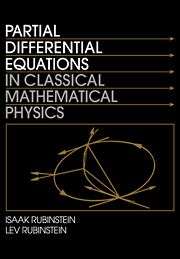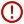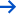Skip to content# Partial Differential Equations in Classical Mathematical Physics

• Date Published: July 1998
• availability: Available
• format: Paperback
• isbn: 9780521558464

## Paperback

### Request inspection copy

Lecturers may request a copy of this title for inspection

Description
Product filter button
Description
Contents
Resources
Courses
• The unique feature of this book is that it considers the theory of partial differential equations in mathematical physics as the language of continuous processes, that is, as an interdisciplinary science that treats the hierarchy of mathematical phenomena as reflections of their physical counterparts. Special attention is drawn to tracing the development of these mathematical phenomena in different natural sciences, with examples drawn from continuum mechanics, electrodynamics, transport phenomena, thermodynamics, and chemical kinetics. At the same time, the authors trace the interrelation between the different types of problems - elliptic, parabolic, and hyperbolic - as the mathematical counterparts of stationary and evolutionary processes. This combination of mathematical comprehensiveness and natural scientific motivation represents a step forward in the presentation of the classical theory of PDEs, one that will be appreciated by both students and researchers alike.

• PDEs are an essential topic in applied maths, natural science and engineering
• Successful hardback edition
• Unique style, employing a motivated approach
• Very experienced authors (father and son team known to most applied mathematicians)

## Reviews & endorsements

'There is no doubt that this is a work of considerable and thorough erudition.' The Times Higher Education Supplement

## Customer reviews

### Not yet reviewed

Be the first to review

Review was not posted due to profanity

×

## , create a review

(If you're not , sign out)

Your review must be a minimum of 12 words.

×

## Product details

• Date Published: July 1998
• format: Paperback
• isbn: 9780521558464
• length: 696 pages
• dimensions: 243 x 169 x 36 mm
• weight: 1.094kg
• contains: 80 b/w illus.
• availability: Available

Preface
1. Introduction
2. Typical equations of mathematical physics. Boundary conditions
3. Cauchy problem for first-order partial differential equations
4. Classification of second-order partial differential equations with linear principal part. Elements of the theory of characteristics
5. Cauchy and mixed problems for the wave equation in R1. Method of travelling waves
6. Cauchy and Goursat problems for a second-order linear hyperbolic equation with two independent variables. Riemann's method
7. Cauchy problem for a 2-dimensional wave equation. The Volterra-D'Adhemar solution
8. Cauchy problem for the wave equation in R3. Methods of averaging and descent. Huygens's principle
9. Basic properties of harmonic functions
10. Green's functions
11. Sequences of harmonic functions. Perron's theorem. Schwarz alternating method
12. Outer boundary-value problems. Elements of potential theory
13. Cauchy problem for heat-conduction equation
14. Maximum principle for parabolic equations
15. Application of Green's formulas. Fundamental identity. Green's functions for Fourier equation
16. Heat potentials
17. Volterra integral equations and their application to solution of boundary-value problems in heat-conduction theory
18. Sequences of parabolic functions
19. Fourier method for bounded regions
20. Integral transform method in unbounded regions
21. Asymptotic expansions. Asymptotic solution of boundary-value problems
Appendix I. Elements of vector analysis
Appendix II. Elements of theory of Bessel functions
Appendix III. Fourier's method and Sturm-Liouville equations
Appendix IV. Fourier integral
Appendix V. Examples of solution of nontrivial engineering and physical problems
References
Index.

• ## Authors

Isaak Rubinstein, Ben-Gurion University of the Negev, Israel

Lev Rubinstein, Hebrew University of Jerusalem

### Sorry, this resource is locked

» Proceed

You are now leaving the Cambridge University Press website. Your eBook purchase and download will be completed by our partner www.ebooks.com. Please see the permission section of the www.ebooks.com catalogue page for details of the print & copy limits on our eBooks.

×You must be signed in to your Cambridge account to turn product stock notifications on or off.

Create a Cambridge account×